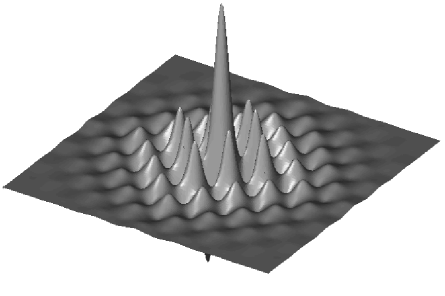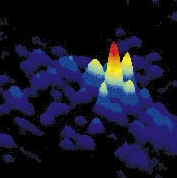## Applied Analysis

The field of Applied Analysis brings together several mathematical topics of great interest and aims at investigating, among others, partial differential equations, probability theory, stochastic partial differential equations, infinite dynamical systems of ordinary differential equations and stochastic analysis. More specifically, the group studies rigorously the dynamics of interfaces for certain reaction-diffusion equations both deterministic and stochastic, sharp interface limits and conservation laws under mean curvature flow. The physical background is diverse, and is stemming mainly from problems of phase transitions, and mathematical physics. Morevover, the group studies large-scale systems of interacting particles and their hydrodynamic limits to model and to rigorously analyze complex systems arising in engineering sciences and physics. The group works towards novel and rigorous results and particular attention is paid to the training of graduate students and postdocs.

## RESEARCH AND DEVELOPMENT ACTIVITIES

Partial differential equations and stochastic differential equations: The interaction between the theory of partial differential equations and probability is very fruitful for research in mathematics, both because of the intrinsic mathematical challenges presented, and because of the range of applications. The study of evolution equations with a stochastic forcing has seen major advances in the recent years. Applications include a variety of important reaction-diffusion equations arising in mathematical physics, but the potential for development of this theory in other applications, such as mathematical finance, mathematical biology and others, is enormous. Research in this direction aims at developing innovative analysis suitable for a rigorous mathematical study of the effects of thermal fluctuations. These include among others the study of stochastic reaction-diffusion equations, motion by mean curvature with random forcing, Malliavin calculus, applications to finance and others.Integrable Systems: We have analyzed so-called "completely integrable" infinite dimensional Hamiltonian systems, in  particular partial differential equations and nonlinear lattices (large systems of ODEs). We have been particularly interested in asymptotic problems like the investigation of long time asymptotics, semiclassical asymptotics, zero dispersion limits and continuum limits of solutions of initial and initial-boundary value problems for nonlinear dispersive partial differential equations and nonlinear lattices, including difficult problems involving instabilities. We have used and extended techniques from PDE theory, complex analysis, harmonic analysis, potential theory and algebraic geometry. Along the way, we have made contributions to the analysis of Riemann-Hilbert factorization problems on the complex plane or a hyperelliptic Riemann surface and the related theory of variational problems for Green potentials with harmonic external fields. In a sense we have worked on a "nonlinear microlocal analysis" that generalizes the classical theory of stationary phase and steepest descent.Large Scale Stochastic Dynamics: Interacting particle systems are models with many degrees of freedom whose stochastic dynamics is prescribed at a microscopic scale. Such models have been very successful in modelling and rigorously analyzing complex systems motivated by engineering sciences, physics, and finance. The aim is to understand and to derive quantitative effective descriptions of phenomena that emerge on the macroscopic scale, and to relate macroscopic observables of these systems to the parameters of their microscopic interactions.Education and Training: The group contributes to the education and training of undergraduate, graduate and post-graduate students as well as of PhD candidates and Postdoctoral researchers.

## PUBLICATIONS

• 2023

• Alikakos N., Geng Z. (2023) On the triple junction problem with general surface tension coefficients, arXiv preprint arXiv:2304.13106
• Antonopoulou D., Farazakis D., Karali G. (2023) Existence of maximal solutions for the financial stochastic Stefan problem of a volatile asset with spread, preprint
• Antonopoulou D., Karali G., D. Li  (2023) Linearized Runge-Kutta methods for the ε-dependent Cahn-Hilliard/Allen-Cahn equation, preprint
• Barbatis, G., Gkikas, K.T.,  Tertikas, A. (2023) Heat and Martin Kernel estimates for Schrödinger operators with critical Hardy potentials. Math. Ann., https://doi.org/10.1007/s00208-023-02693-9
• Filippas, S., Tersenov, A. (2023) Estimates on the velocity of a rigid body moving in a fluid, arXiv preprint arXiv:2304.11887.

• 2022
• 2021
• 2020
• 2019
• 2018
• 2017
• 2016
• 2015
• 2014
• 2013

RESEARCHERS